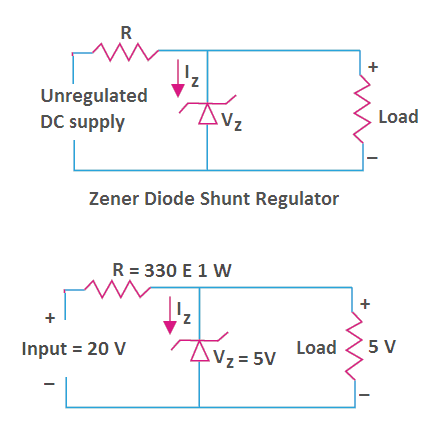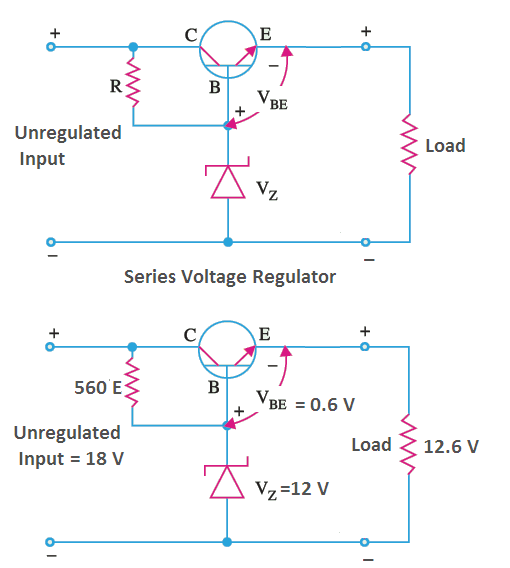# Zener Diode as Voltage Regulator

Zener diode is a crystal diode having unusual reverse current characteristics which are particularly suitable for voltage regulating purposes. When a reverse voltage applied to a zener diode is increased, a value is reached at which the current increases greatly from its normal reverse value.

This voltage is known as zener voltage (Vz) or breakdown voltage and the reverse current as zener current (Iz).# Zener Diode as Voltage Regulator

The Figure shows the fuction of zener diode as voltage regulator. The diode is selected in such a way that its breakdown voltage is equal to the desired output voltage.  When the input voltage is less than the breakdown voltage (Vz), very small current flows through the diode and the whole input appears across the output terminals.

But when the input voltage reaches the breakdown voltage, breakdown takes place and current through the diode (Iz) is adjusted itself such that the voltage across the zener diode remains constant. As long as the input voltage is greater than zener voltage (Vz), the zener operates in breakdown region and maintains a constant voltage across the load.

This circuit cannot be used efficiently for heavy load currents. If the load current required is high, the power loss in series resistance will be quite high and the efficiency of supply becomes very small.

## Transistor Series Voltage RegulatorWhen load current requirement is high a zener controlled transistor is used for obtaining a constant voltage and this circuit is known as the transistor series voltage regulator.

Here, the transistor behaves like a variable resistor whose resistance is determined by the base current. In this circuit, as long as input voltage is greater than zener voltage, the zener operates in breakdown region and the output voltage remains constant ( = Vz + Vbe).

Vbe is quite small as compared to Vz. Moreover, Vbe varies little with emitter or collector current (load current) as it is clear from input characteristics of the transistor. Therefore, the output voltage is substantially constant.

## Electronics | All Posts

© https://yourelectricalguide.com/ zener diode as voltage regulator.

### 2 thoughts on “Zener Diode as Voltage Regulator”

1.why the resistor is used across transistor

1.Ajay Sharma

Here, the resistor is used to providing some positive voltage at the base of the transistor (i.e. biasing). In the absence of it, the transistor will not conduct.